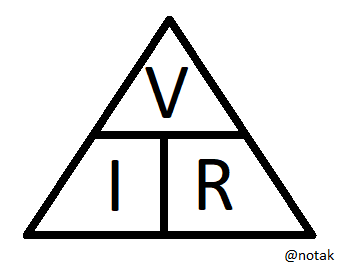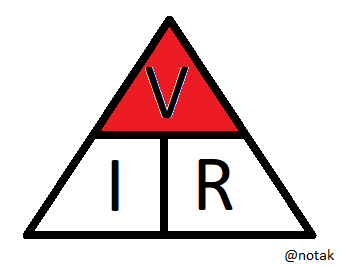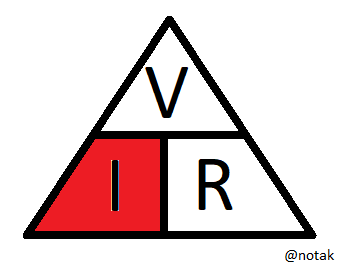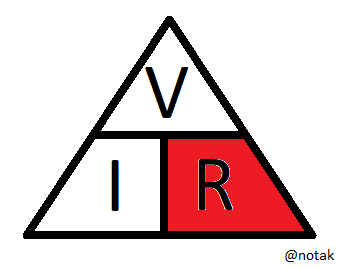# Ohms Law for Beginners (voltage and current)

in #stemgeeks2 years ago (edited)

When I was growing up, I joined an amateur radio class, and we needed to pass our radio course to get our license. I haven't revisited that hobby, but hopefully one day I will find the time.

The benefit of learning electronics with that group, was there was no fancy calculus, or hard maths, it was down to good practical ways of finding out what you needed to do.

They explained ohms law so easily, I thought I would share the technique, you don't even need to know really how to grasp algebra.

# So what is ohms law?

Ohms law is what governs voltage, electric current, and resistance. Its the law that relates all of these together. A quick summary of each of these is below:

Voltage - This is how strong the electricity is, in the water analogy its like the water pressure.
Current - This is how much electrons are flowing - In the water analogy its how much water is flowing.
Resistance - This is how much the wires (or other components) resists electricity, much like having a pinch point in pipes in the water analogy.

So the formula for ohms law is V = I X R

V = Voltage in volts
I = Current in Amps
R = Resistance in Ohms

# What is an easy way to remember it

So I like to refer to the ohms law triangle, I have drawn this below:How we use this triangle is simple, if you need to find Voltage you put your finger over the Voltage, and you can see I is next to R, this means you multiply them to find Voltage as shown below:i.e. V = I X R

If you need to find Current you put your finger over the I and that leaves V over R, as shown below:I.e. I = V / R

And finally if you need to find resistance you put your finger over the R, and this leaves you with V over I as shown below:i.e. R = V/ I

# Other useful tricks

The other thing I find useful, is in using consistent units, for example:

If you are using low current devices, then the formula works if you use milliamps (i.e. 10^-3 Amps), volts, but also kiloOhms (10^3 ohms)

If you are using high voltage, low amperage, you can use Kilovolts, amps, and kiloOhms

There are lots of other combinations too.

I hope this gives you an easy way to understand ohms law, if you even need it.

Sort:

Congratulations @notak! You have completed the following achievement on the Hive blockchain and have been rewarded with new badge(s) :You got more than 200 replies.Your next target is to reach 300 replies.

If you no longer want to receive notifications, reply to this comment with the word `STOP`